# 定时器

## 时钟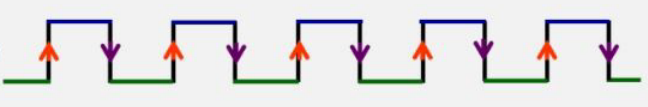## 定时器寄存器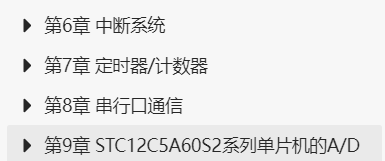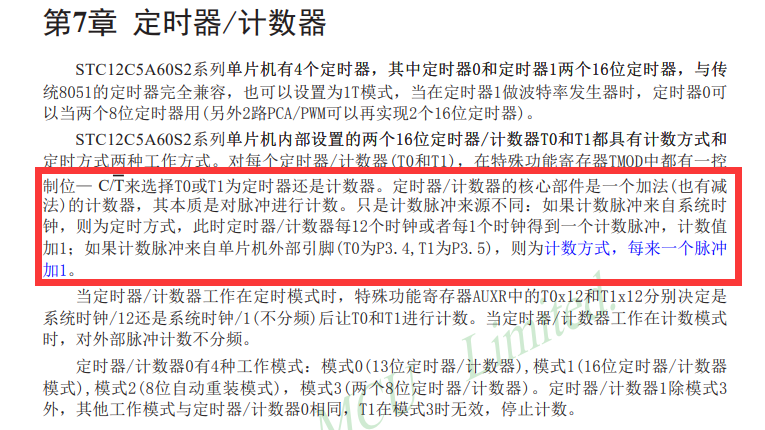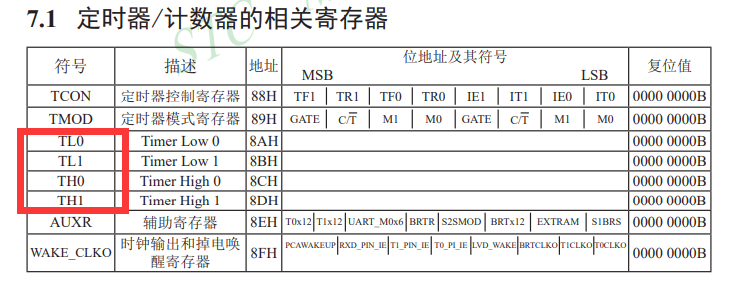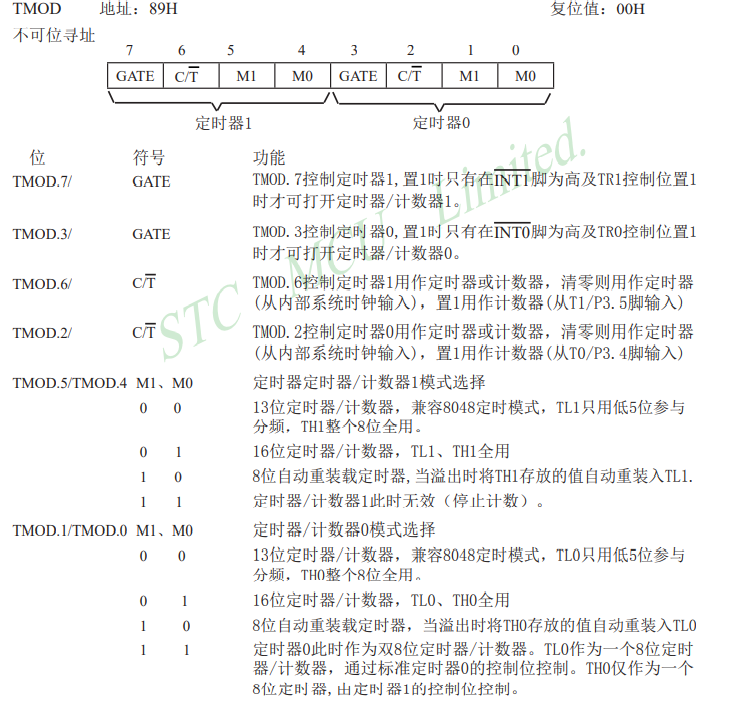### 10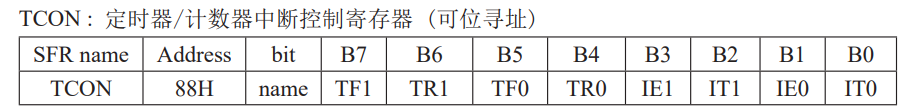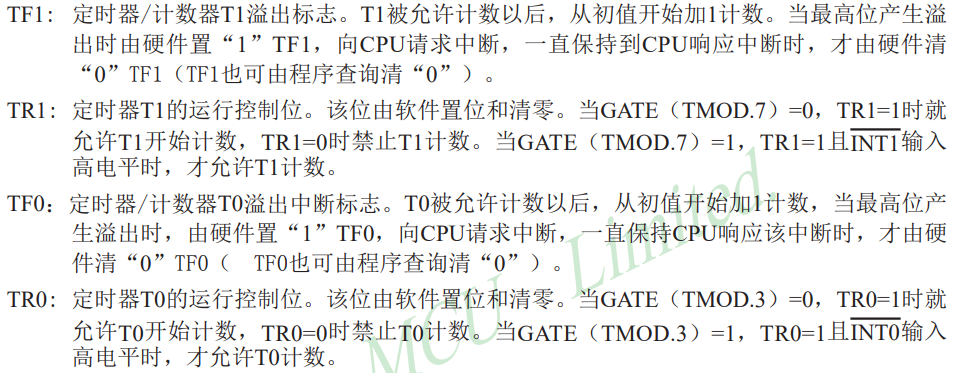## 代码例程

//所以，我们要开启定时器计时，就对这些寄存器配置就行
void Init(void) {
    TCOM = 0x01;    //把定时器1配置成01的模式
    TR0 = 1;        //允许定时器1计数
    //定时器计数的初始化就完成了，我们可以通过读取TH和TL的值来判断代码运行时间
}

# 定时器中断

## 中断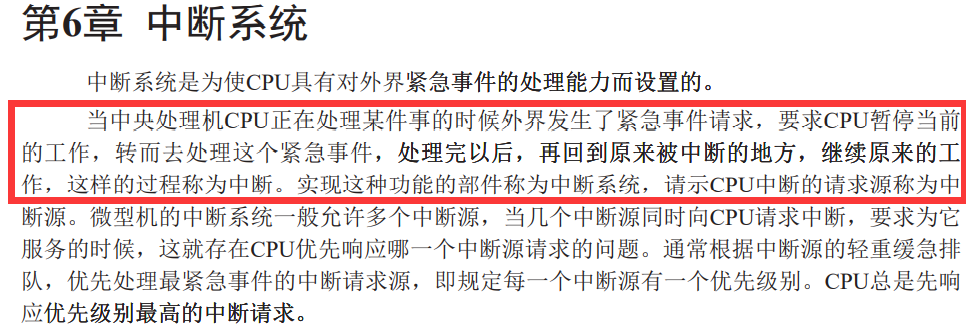## 定时器中断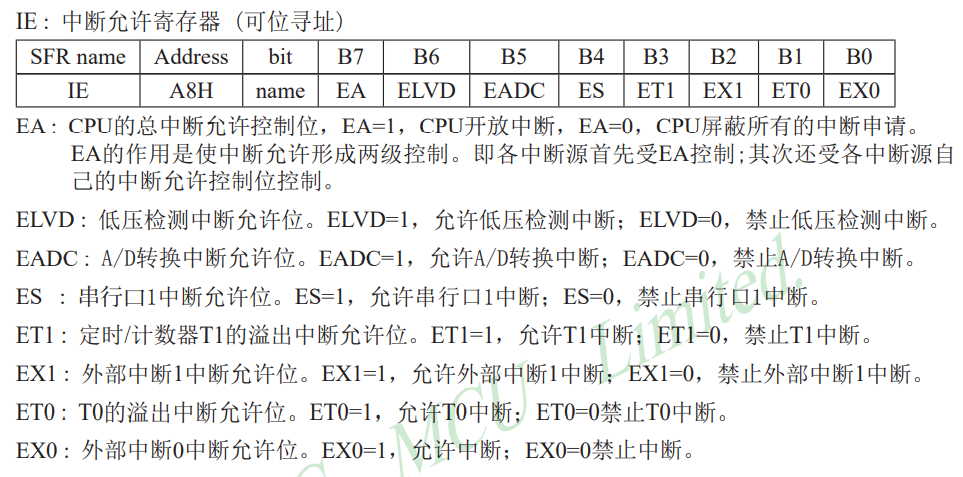## 代码例程

void Init(void) {
    TCOM = 0x01;
    TR0 = 1;
    ET0 = 1;        //允许定时器中断
    EA = 1;            //打开总中断
}

//打开了中断，那我们怎么跳转到中断事件去呢？可以通过中断函数
void Int0(void) interrupt 1 {    //中断函数是特殊的函数，不需要在main函数之中调用，并且需要通过interrupt来标注这是一个中断函数，后面的数字代表了不同的中断函数，后面会讲。
    //这儿就可以写我们需要做的中断事件。
}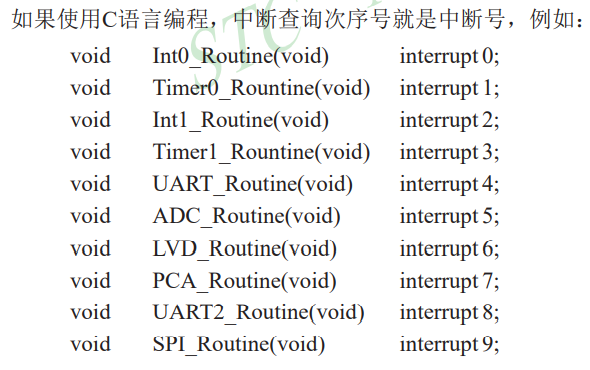## 代码例程

//当使用模式 1 0 时
//我们只赋值一次就可以，每次溢出后TL的值会变成我们设置好的TH的值
void Init(void) {
    TCOM = 0x02;
    TH0 = 10;    //每次TL溢出后讲TH的值装进去
    TL0 = 10;    //TL一开始就从10开始计数人为的控制进入中断的间隔时间
    TR0 = 1;
    ET0 = 1;
    EA = 1;
}

void Int0(void) interrupt 1 {
}

//当使用模式 0 1 时
//我们只赋值一次就可以，每次溢出后TL的值会变成我们设置好的TH的值
void Init(void) {
    TCOM = 0x01;
    TH0 = 10;    //TH一开始就从10开始计数人为的控制进入中断的间隔时间
    TL0 = 10;    //TL一开始就从10开始计数人为的控制进入中断的间隔时间
    TR0 = 1;
    ET0 = 1;
    EA = 1;
}

void Int0(void) interrupt 1 {
    TH0 = 10;    //由于不会自动的赋值，所以我们需要在每次进入中断函数（也就是刚刚好溢出时）重新赋值
    TL0 = 10;
}

# 定时器流水灯实现

#include <STC12C5A60S2.h>

unsigned int nTimer = 0;            //定义一个变量作为后面计时使用

void main(void) {
    unsigned char i = 0;        //定义一个变量作为后面流水使用

    TMOD = 0x01;                //定时器模式设置成定时器1 01模式
    TH0 = -9;                    //通过计算我们可以得到 115200/12/256/9 = 400，1/400 = 0.0025s定时器溢出，进入一次中断
    ET0 = 1;                    //打开定时器中断
    TR0 = 1;                    //允许中断计数
    EA = 1;                        //打开总中断
    while (1) {
        if(nTimer >= 200) {        //当定时器溢出200次时流水一次，流水灯以200*0.0025 = 0.5s速度流水
            P0 = 0x01 << i++;    //流水灯流水
            i &= 7;                //当i>=8时，将i清零
            nTimer = 0;            //次数清零
        }
    }
}

void Int0(void) interrupt 1 {    //中断函数
    TH0 = -9;                    //重新赋初值
    nTimer++;                    //每次进入中断函数计数加1
}

# 定时器按键实现

#include <STC12C5A60S2.h>

unsigned int nTimer = 0;
unsigned char cKey, cKeyCode;
unsigned int nDelayKey, nLED_Time = 200;
bit bLoose, bStop;

void DisposeKey(void);    //按键函数

void main(void) {
    unsigned char i = 0;

    TMOD = 0x01;
    TH0 = -9;
    ET0 = 1;
    TR0 = 1;
    EA = 1;
    while (1) {
        if (nTimer >= nLED_Time) {
            if (bStop == 0) P0 = 0x01 << i++;
            i &= 7;
            nTimer = 0;
        }
        if (cKeyCode != 0) DisposeKey();    //判断按键按下后执行按键函数
    }
}

void Int0(void) interrupt 1 {
    TH0 = -9;
    nTimer++;
    if (nDelayKey == 0) {    //当没有按键按下时，此时恒为0
        cKey = P2 & 0x07    //将按键的值保存在cKey中
        if (cKey != 0x07) nDelayKey = 4;    //当按键按下后，值不等于0x70，将nDelayKey赋4用来消抖
        else bLoose = 0;    //松开按键后清零松手标志
    } else {
        if (--nDelayKey == 0) {    //定时器进入四次，相当于10ms按键消抖
            cKeyCode = P2 & 0x07;    //再次存入按键的值
            if (cKeyCode != cKey) cKeyCode = 0;    //如果前后两次按键值不相等，就证明按键没有按下，将按键值清零
        }
    }
}

void DisposeKey(void) {
    if(bLoose == 0) {    //判断是否松手，没有松手就只会执行一次按键的作用
        if (cKeyCode == 6) {    //按键具体值
            if (nLED_Time <= 400) nLED_Time += 40;    //流水速度加快
        } else if (cKeyCode == 5) {
            if (nLED_Time >= 40) nLED_Time -= 40;    //流水速度减慢
        } else if (cKeyCode == 3) bStop = !bStop;    //流水停止
        bLoose = 1;    //表示此次按键按下按键作用已经执行了一次了
    }
    cKeyCode = 0;    //对按键进行清零
}